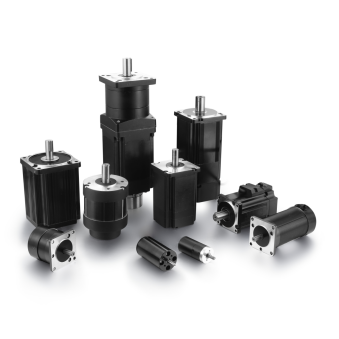## What are the characteristics of high-efficiency motors —— BG Motor

NEWS    |    Dec 22, 2022

To understand the concept of an efficient motor, you must first know how to calculate efficiency and the losses associated with the motor components themselves.

The final measured efficiency of electric motors is calculated based only on the application-specific elements in which they are used. For the motor itself, with no load, the manufacturer provides a rating based on a standard formula. To understand efficient motors, you just need to know what makes them special.

But first, let's look at the basic concept used to explain motor efficiency, which is the ratio between shaft output power and electrical input power. Shaft output can be measured in horsepower or watts. For the purposes of this article, we'll be using watts. The most commonly used formula is the simple one mentioned above:

ηm = P out / P in

where

ηm = motor efficiency

P out = shaft power out (Watts)

P in = electric power to the motor (Watts)

Once you have used this formula and found your motor efficiency, motor efficiency drops based on many known factors, where power is lost as current through the motor encounters various resistances. These losses may include wiring and its resistance, iron losses due to magnetic events, and heat losses.

The electrical power lost in the primary rotor and secondary stator windings is called resistive losses (or copper losses, as they are based on the characteristics of the wires used, including their diameter and length). Both primary and secondary resistive losses vary with load and are proportional to the square of the current. E.g:

Pcl = R I2

where

Pcl = stator winding, copper loss (W)

R = resistance (?)

I = current (Amp)

Other losses include iron losses, as described above. These losses are a result of the magnetic energy dissipated as the motor's magnetic field acts on the stator core. Other factors involved include mechanical losses, which involve friction and stray losses in the motor bearings.

The biggest culprit of stray losses is the harmonic energy generated by the motor when it is running under load. Load affects shaft power output, which is why it's impossible to discuss in a general article like this one. but basically

In addition, these losses are dissipated in the form of currents in the windings, harmonic flux components in iron components, and leakage in the laminated core.

Generally, we recommend judging whether the motor is a high-efficiency motor from these characteristics:1.Save energy and reduce long-term operating costs. It is very suitable for textiles, fans, water pumps, and compressors. The motor purchase cost can be recovered by saving electricity for one year;

2. The asynchronous motor can be completely replaced by starting directly or adjusting the speed with a frequency converter;

3. The rare earth permanent magnet high-efficiency energy-saving motor itself can save more than 15℅ of electric energy than ordinary motors;

4. The power factor of the motor is close to 1, which improves the quality factor of the power grid without adding a power factor compensator;

3. The motor current is small, which saves power transmission and distribution capacity and prolongs the overall operating life of the system;

4. Power-saving budget: Taking a 55Kw motor as an example, a high-efficiency motor saves 15% of electricity compared with a general motor, and the electricity bill is calculated at 0.5 yuan per kilowatt-hour.

5. The cost of replacing the motor can be recovered by saving electricity within one year of using the energy-saving motor.

If you don't know the characteristics of high-efficiency motors or whether the motor can be used in your product, you can contact our salesmen, and they will give you professional answers.BACK